# 一 随机森林简介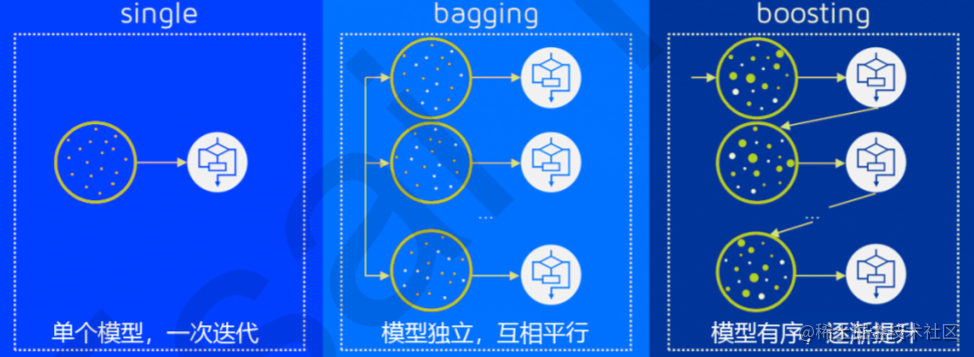装袋法的核心思想是构建多个相互独立的评估器，然后对其预测进行平均或多数表决原则来决定集成评估器的结 果。装袋法的代表模型就是随机森林。 提升法中，基评估器是相关的，是按顺序一一构建的。其核心思想是结合弱评估器的力量一次次对难以评估的样本 进行预测，从而构成一个强评估器。提升法的代表模型有Adaboost和梯度提升树。

# 二 重要参数

## 2.2 RandomForestClassifier VS DecisionTreeClassifier

``````from sklearn.tree import DecisionTreeClassifier
from sklearn.ensemble import RandomForestClassifier

wine.data
wine.target

#同时使用随机森林和决策树进行结果比较
from sklearn.model_selection import train_test_split
x_train,x_test,y_train,y_test=train_test_split(wine.data,wine.target,test_size=0.3)

clf=DecisionTreeClassifier(random_state=200)
rfc=RandomForestClassifier(random_state=200)
clf=clf.fit(x_train,y_train)
rfc=rfc.fit(x_train,y_train)
score_c=clf.score(x_test,y_test)
score_r=rfc.score(x_test,y_test)

print('Tree Score:{}'.format(score_c), ' \n'  'Random Forest Score:{}'.format(score_r))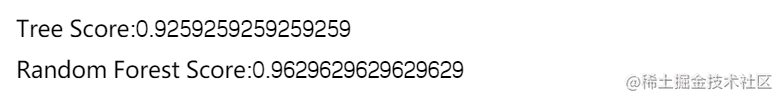随机森林结果高于决策树结果

## 2.3 再次使用交叉验证进行比较(cross_val_score)

``````from sklearn.model_selection import cross_val_score
import matplotlib.pyplot as plt

rfc=RandomForestClassifier(n_estimators=30)
rfc_s=cross_val_score(rfc,wine.data,wine.target,cv=10)

clf=DecisionTreeClassifier()
clf_s=cross_val_score(clf,wine.data,wine.target,cv=10)
plt.figure(figsize=(10,5))
plt.plot(range(1,11),rfc_s,label='RandomForest')
plt.plot(range(1,11),clf_s,label='Decision Tree')
plt.title('RandomForest VS Decision Tree')
plt.legend()
plt.show()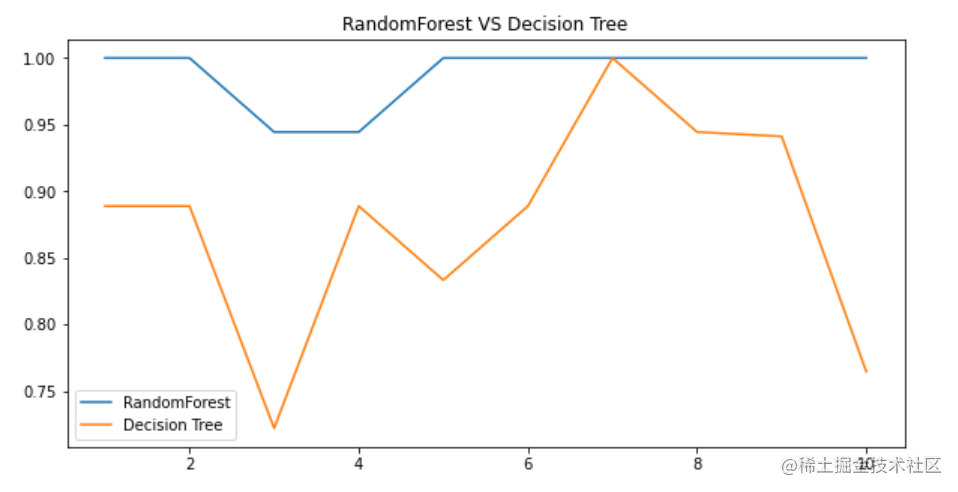10次交叉验证下，虽然决策树的结果有时和随机森林相同，但是大总体上，随机森林结果显著优于决策树。

# 三 袋装法

## 3.1 bootstrap&oob_score

``````rfc=RandomForestClassifier(n_estimators=30,oob_score=True)
rfc=rfc.fit(wine.data,wine.target)
rfc.oob_score_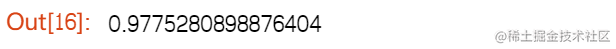# 四 重要属性和接口

``````rfc =RandomForestClassifier(n_estimators=25)
rfc=rfc.fit(x_train,y_train)

#预测结果和标签相比的正确率
print('Random Forest Score:{}'.format(rfc.score(x_test,y_test)))
print('-'*100)
#特征重要性
print('特征重要性:{}'.format([*zip(wine.feature_names,rfc.feature_importances_)]))
print('-'*100)
#每个样本在每棵树中叶子节点的索引
print('每个样本在每棵树中叶子节点的索引:{}'.format(rfc.apply(x_test)[:2]))
print('-'*100)
#测试集预测的标签
print('测试集预测的标签:{}'.format(rfc.predict(x_test)))
print('-'*100)
#每个样本被分到每个标签的概率
print('每个样本被分到每个标签的概率:{}'.format(rfc.predict_proba(x_test)[:5]))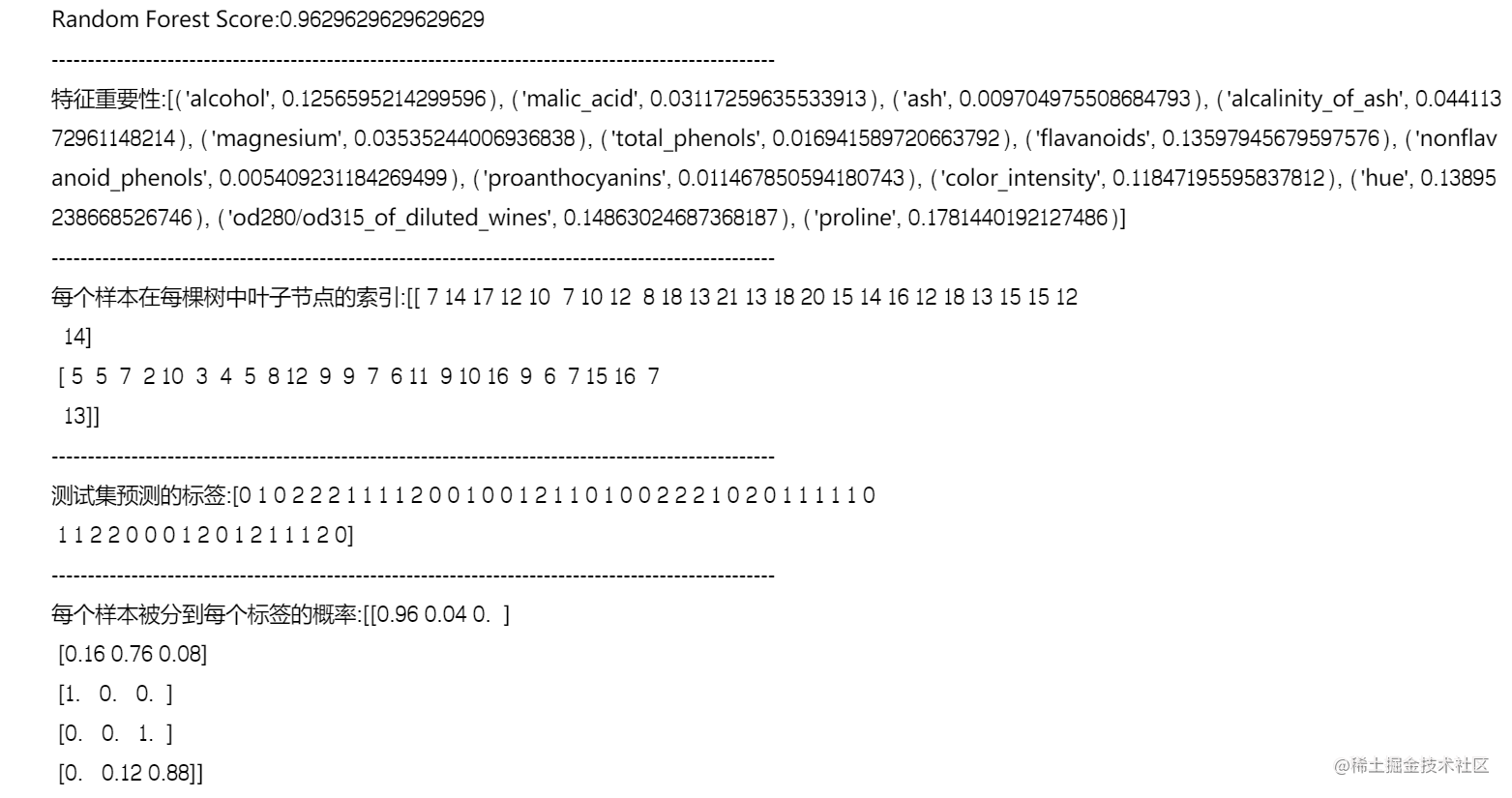**在使用随机森林之前，一定要检查，用来组成随机 森林的分类树们是否都有至少50%的预测正确率。